# LFSR¶

## Installation¶

Requirement : numpy, matplotlib

With pip:

```pip install pylfsr
```

Build from source

Download the repository or clone it with git, after cd in directory build it from source with

```python setup.py install
```

# Examples¶

## Example 1: 5-bit LFSR with feedback polynomial: x5+ x2+1¶

default feedback polynomial is p(x) = x5+ x2+ 1

```# import LFSR
import numpy as np
from pylfsr import LFSR

L = LFSR()

# print the info
L.info()

5 bit LFSR with feedback polynomial  x^5 + x^2 + 1
Expected Period (if polynomial is primitive) =  31
Current :
State        :  [1 1 1 1 1]
Count        :  0
Output bit   : -1
feedback bit : -1
```
```L.next()
L.runKCycle(10)
L.runFullCycle()
L.info()
```

## Example 2: 5-bit LFSR with custum state and feedback polynomial¶

```state = [0,0,0,1,0]
fpoly = [5,4,3,2]
L = LFSR(fpoly=fpoly,initstate =state, verbose=True)
L.info()
tempseq = L.runKCycle(10)
L.set(fpoly=[5,3])
```

## Example 3: 23-bit LFSR: x23+ x18+1¶

```L = LFSR(fpoly=[23,18],initstate ='random',verbose=True)
L.info()
L.runKCycle(10)
L.info()
seq = L.seq
```

## Example 4: 23-bit LFSR: x23+ x5+1¶

```fpoly = [23,5]
L1 = LFSR(fpoly=fpoly,initstate ='ones', verbose=False)
L1.info()
```
```23 bit LFSR with feedback polynomial  x^23 + x^5 + 1
Expected Period (if polynomial is primitive) =  8388607
Current :
State        :  [1 1 1 1 1 1 1 1 1 1 1 1 1 1 1 1 1 1 1 1 1 1 1]
Count        :  0
Output bit   :  -1
feedback bit :  -1
```

## Example 5: Plotting LFSR with pylsr¶

Each LFSR can be visualize as it in current state by using .Viz() method

```L = LFSR(initstate=[1,1,0,1,1],fpoly=[5,2])
L.runKCycle(15)
L.Viz(title='R1')
```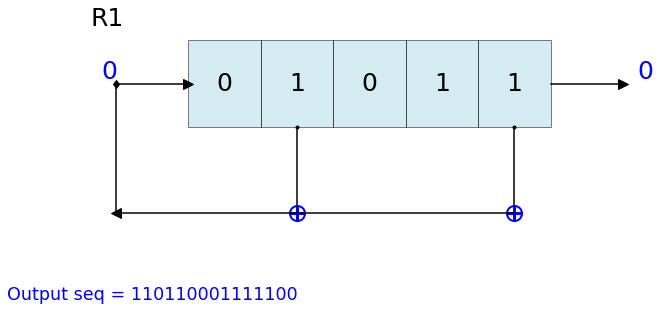## Example 6: Dynamic visualization of LFSR - Animation*¶

```%matplotlib notebook
L = LFSR(initstate=[1,0,1,0,1],fpoly=[5,4,3,2],counter_start_zero=False)
```
```fig, ax = plt.subplots(figsize=(8,3))
for _ in range(35):
ax.clear()
L.Viz(ax=ax, title='R1')
plt.ylim([-0.1,None])
#plt.tight_layout()
L.next()
fig.canvas.draw()
plt.pause(0.1)
```## Setting clock start:¶

Initial output bit An argument counter_start_zero can be used to initialize the output bit. * If counter_start_zero=True (default), the output bit is initialize by -1, to illustrate that No clock is provided yet.

In this case, cout (counter) starts with 0. The first output is not computed until first cylce is executed, such as by executing .next(), .runFullCycle, etc
• If counter_start_zero=False, the output bit is initialize by the last bit of register. In one sense, first clock cycle is executed. This is why, in this case, cout (counter) starts with 1.

In both cases counter_start_zero =True or False, the L.seq will be same, the only difference is the total number of output bits produced after N-cycles, i.e. when setting counter_start_zero = False, there will be one extra bit, since first bit was already computed. To understand this, look at following two examples. counter_start_zero=True can be seen as dealyed response by one bit.

## Example 7.1: Visualize, 3-bit LFSR at each step, with default counter_start_zero = True¶

```state = [1,1,1]
fpoly = [3,2]
L = LFSR(initstate=state,fpoly=fpoly)
print('count \t state \t\toutbit \t seq')
print('-'*50)
for _ in range(15):
print(L.count,L.state,'',L.outbit,L.seq,sep='\t')
L.next()
print('-'*50)
print('Output: ',L.seq)
```
```count          state          outbit   seq
--------------------------------------------------
0             [1 1 1]         -1      [-1]
1             [0 1 1]         1       
2             [0 0 1]         1       [1 1]
3             [1 0 0]         1       [1 1 1]
4             [0 1 0]         0       [1 1 1 0]
5             [1 0 1]         0       [1 1 1 0 0]
6             [1 1 0]         1       [1 1 1 0 0 1]
7             [1 1 1]         0       [1 1 1 0 0 1 0]
8             [0 1 1]         1       [1 1 1 0 0 1 0 1]
9             [0 0 1]         1       [1 1 1 0 0 1 0 1 1]
10            [1 0 0]         1       [1 1 1 0 0 1 0 1 1 1]
11            [0 1 0]         0       [1 1 1 0 0 1 0 1 1 1 0]
12            [1 0 1]         0       [1 1 1 0 0 1 0 1 1 1 0 0]
13            [1 1 0]         1       [1 1 1 0 0 1 0 1 1 1 0 0 1]
14            [1 1 1]         0       [1 1 1 0 0 1 0 1 1 1 0 0 1 0]
--------------------------------------------------
Output:  [1 1 1 0 0 1 0 1 1 1 0 0 1 0 1]
```

## Example 7.2: Visualize, 3-bit LFSR at each step, with counter_start_zero = False¶

```state = [1,1,1]
fpoly = [3,2]
L = LFSR(initstate=state,fpoly=fpoly,counter_start_zero=False)
print('count \t state \t\toutbit \t seq')
print('-'*50)
for _ in range(15):
print(L.count,L.state,'',L.outbit,L.seq,sep='\t')
L.next()
print('-'*50)
print('Output: ',L.seq)
```
```count          state          outbit   seq
--------------------------------------------------
1     [1 1 1]         1       
2     [0 1 1]         1       [1 1]
3     [0 0 1]         1       [1 1 1]
4     [1 0 0]         0       [1 1 1 0]
5     [0 1 0]         0       [1 1 1 0 0]
6     [1 0 1]         1       [1 1 1 0 0 1]
7     [1 1 0]         0       [1 1 1 0 0 1 0]
8     [1 1 1]         1       [1 1 1 0 0 1 0 1]
9     [0 1 1]         1       [1 1 1 0 0 1 0 1 1]
10    [0 0 1]         1       [1 1 1 0 0 1 0 1 1 1]
11    [1 0 0]         0       [1 1 1 0 0 1 0 1 1 1 0]
12    [0 1 0]         0       [1 1 1 0 0 1 0 1 1 1 0 0]
13    [1 0 1]         1       [1 1 1 0 0 1 0 1 1 1 0 0 1]
14    [1 1 0]         0       [1 1 1 0 0 1 0 1 1 1 0 0 1 0]
--------------------------------------------------
Output:  [1 1 1 0 0 1 0 1 1 1 0 0 1 0 1]
```

## LFSR Properties:: Test 3+1 properties of LFSR¶

Using test_properties(verbose=1) method, it we can test if LSFR set be state and polynomial setisfies the following properites in addition to periodicity (period T = 2^M -1) for M-bit LFSR * (1) Balance Property * (2) Runlength Property * (3) Autocorrelation Property

## Example 8.1: test [5,3], for 5-bit LFSR, which we know is a primitive polynomial¶

```state = [1,1,1,1,0]
fpoly = [5,3]
L = LFSR(initstate=state,fpoly=fpoly)
result  = L.test_properties(verbose=2)
```
```1. Periodicity
------------------
- Expected period = 2^M-1 = 31
- Pass?:  True

2. Balance Property
-------------------
- Number of 1s = Number of 0s+1 (in a period): (N1s,N0s) =  (16, 15)
- Pass?:  True

3. Runlength Property
-------------------
- Number of Runs in a period should be of specific order, e.g. [4,2,1,1]
- Runs:  [8 4 2 1 1]
- Pass?:  True

4. Autocorrelation Property
-------------------
- Autocorrelation of a period should be noise-like, specifically, 1 at k=0, -1/m everywhere else
- Pass?:  True

==================
Passed all the tests
==================
```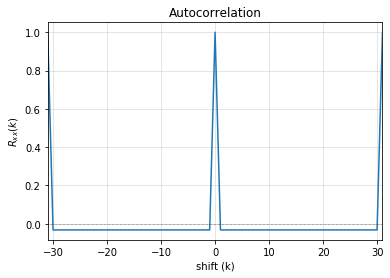## Example 8.2: test [5,1], for 5-bit LFSR, which we know is *NOT* a primitive polynomial¶

```state = [1,1,1,1,0]
fpoly = [5,1]
L = LFSR(initstate=state,fpoly=fpoly)
result  = L.test_properties(verbose=2)
```
```1. Periodicity
------------------
- Expected period = 2^M-1 = 31
- Pass?:  False

2. Balance Property
-------------------
- Number of 1s = Number of 0s+1 (in a period): (N1s,N0s) =  (17, 14)
- Pass?:  False

3. Runlength Property
-------------------
- Number of Runs in a period should be of specific order, e.g. [4,2,1,1]
- Runs:  [10  2  1  1  2]
- Pass?:  False

4. Autocorrelation Property
-------------------
- Autocorrelation of a period should be noise-like, specifically, 1 at k=0, -1/m everywhere else
- Pass?:  False

==================
Failed one or more tests, check if feedback polynomial is primitive polynomial
==================
```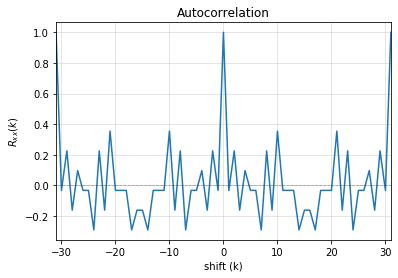## Example 8.3: test individual properties¶

```state = [1,1,1,1,1]
fpoly = [5,4,3,2]
L = LFSR(initstate=state,fpoly=fpoly)

# get one full period
p = L.getFullPeriod()

L.balance_property(p.copy())
L.runlength_property(p.copy())
L.autocorr_property(p.copy())
```

## Feedback (Primitive) Polynomials:¶

A primitive polynomial is is irreducible, and not trivial to derive. A list of primitive polynomials upto 32 degree can be found at Ref, which is not an exhaustive list. Since for each primitive polynomial, an image replica (which is also primitive) can be computed easily list include half of polynomials for each degree and other half can be compputed by get_Ifpoly() method, see example 7.2

## Example 9.1: Get a list of feedback polynomials for a m-bit LFSR¶

```L = LFSR()
# list of 5-bit feedback polynomials
fpolys = L.get_fpolyList(m=5)
[[5, 2], [5, 4, 2, 1], [5, 4, 3, 2]]

# list of all feedback polynomials as a dictionary
fpolyDict = L.get_fpolyList()
```

## Example 9.2: Get a image replica of a feedback polynomial¶

Image replica of a primitive polynomial is a primitive polynomial, hence a valid feedback polynomial for LFSR For m-bit primitive polynomial p(x) = x^m + x^k + .. + 1, a image replica is ip(x) = x^(-m)p(x) where 0 < k < m

```L = LFSR()
L.get_Ifpoly([5,3])
[5, 2]
```
```L.get_Ifpoly([5,4,3,2])
[5, 3, 2, 1]
```

## Example 9.3: Changing feedback polynomial in between¶

After generating some bits from an LFSR, a feedback polynomial can be changed keeping the current state as intial state and generate the new sequece.

```L = LFSR(fpoly=[23,18],initstate ='ones')
seq0 = L.runKCycle(10)

# Change after 10 clocks
L.changeFpoly(newfpoly =[23,14],reset=False)
seq1 = L.runKCycle(20)

# Change after 20 clocks
L.changeFpoly(newfpoly =[23,9],reset=False)
seq2 = L.runKCycle(20)
```

# Generators¶

## A5/1 GSM Stream cipher generator¶```import numpy as np
import matplotlib.pyplot as plt
from pylfsr import A5_1

A5 = A5_1(key='random')
print('key: ',A5.key)
A5.R1.Viz(title='R1')
A5.R2.Viz(title='R2')
A5.R3.Viz(title='R3')

print('key: ',A5.key)
print()
print('count \t cbit\t\tclk\t R1_R2_R3\toutbit \t seq')
print('-'*80)
for _ in range(15):
print(A5.count,A5.getCbits(),A5.clock_bit,A5.getLastbits(),A5.outbit,A5.getSeq(),sep='\t')
A5.next()
print('-'*80)
print('Output: ',A5.seq)

A5.runKCycle(1000)
A5.getSeq()
```

## Enhanced A5/1¶

Reference Article: Enhancement of A5/1: https://doi.org/10.1109/ETNCC.2011.5958486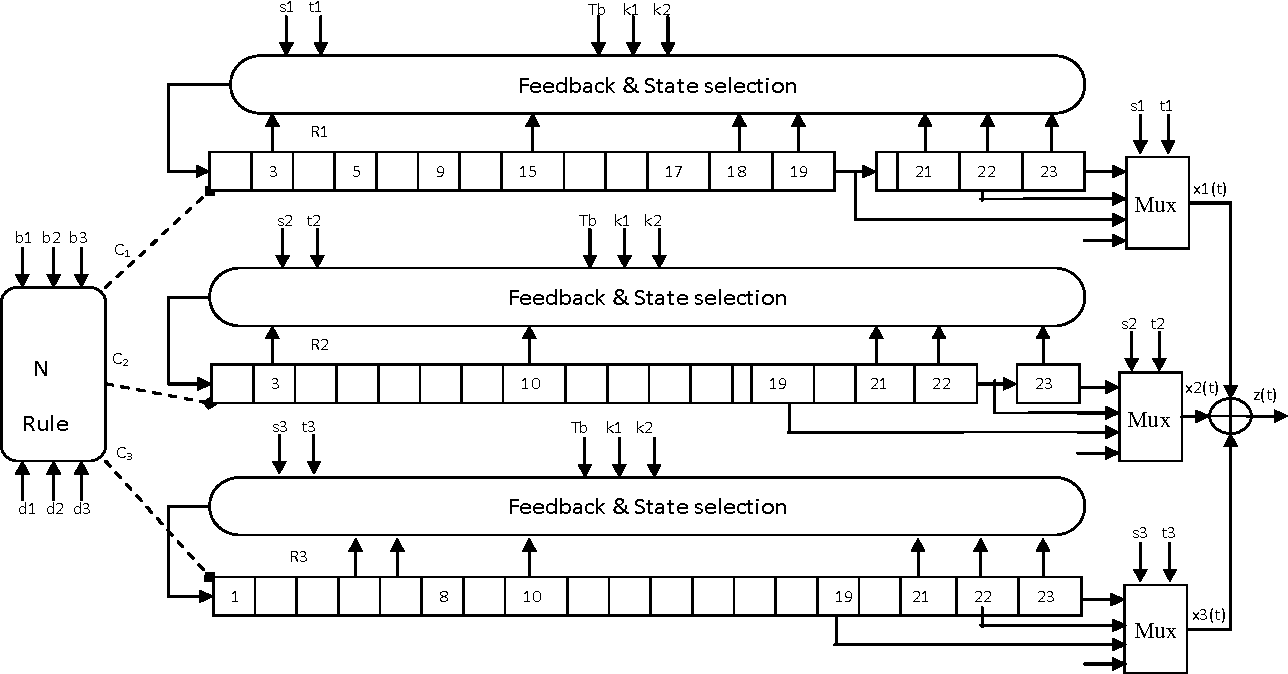```# Three LFSRs initialzed with 'ones' though they are intialized with encription key
R1 = LFSR(fpoly = [19,18,17,14])
R2 = LFSR(fpoly = [23,22,21,8])
R3 = LFSR(fpoly = [22,21])

# clocking bits
b1 = R1.state
b2 = R3.state
b3 = R3.state
```

## Geffe Generator¶

Ref: Schneier, Bruce. Applied cryptography: protocols, algorithms, and source code in C. john wiley & sons, 2007.
Chaper 16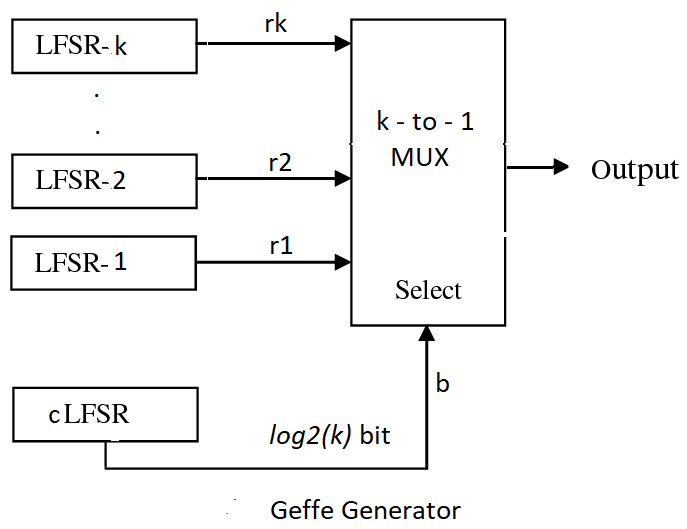```import numpy as np
import matplotlib.pyplot as plt
from pylfsr import Geffe, LFSR

kLFSR = [LFSR(initstate='random') for _ in range(8)]  # List of 8 5-bit LFSRs with default feedback polynomial and random initial state
cLFSR = LFSR(initstate='random')                      # A 5-bit LFSR with for selecting one of 8 output at a time

GG = Geffe(kLFSR_list=kLFSR, cLFSR=cLFSR)

print('key: ',GG.getState())
print()
for _ in range(50):
print(GG.count,GG.m_count,GG.outbit_k,GG.sel_k,GG.outbit,GG.getSeq(),sep='\t')
GG.next()

GG.runKCycle(1000)
GG.getSeq()
```